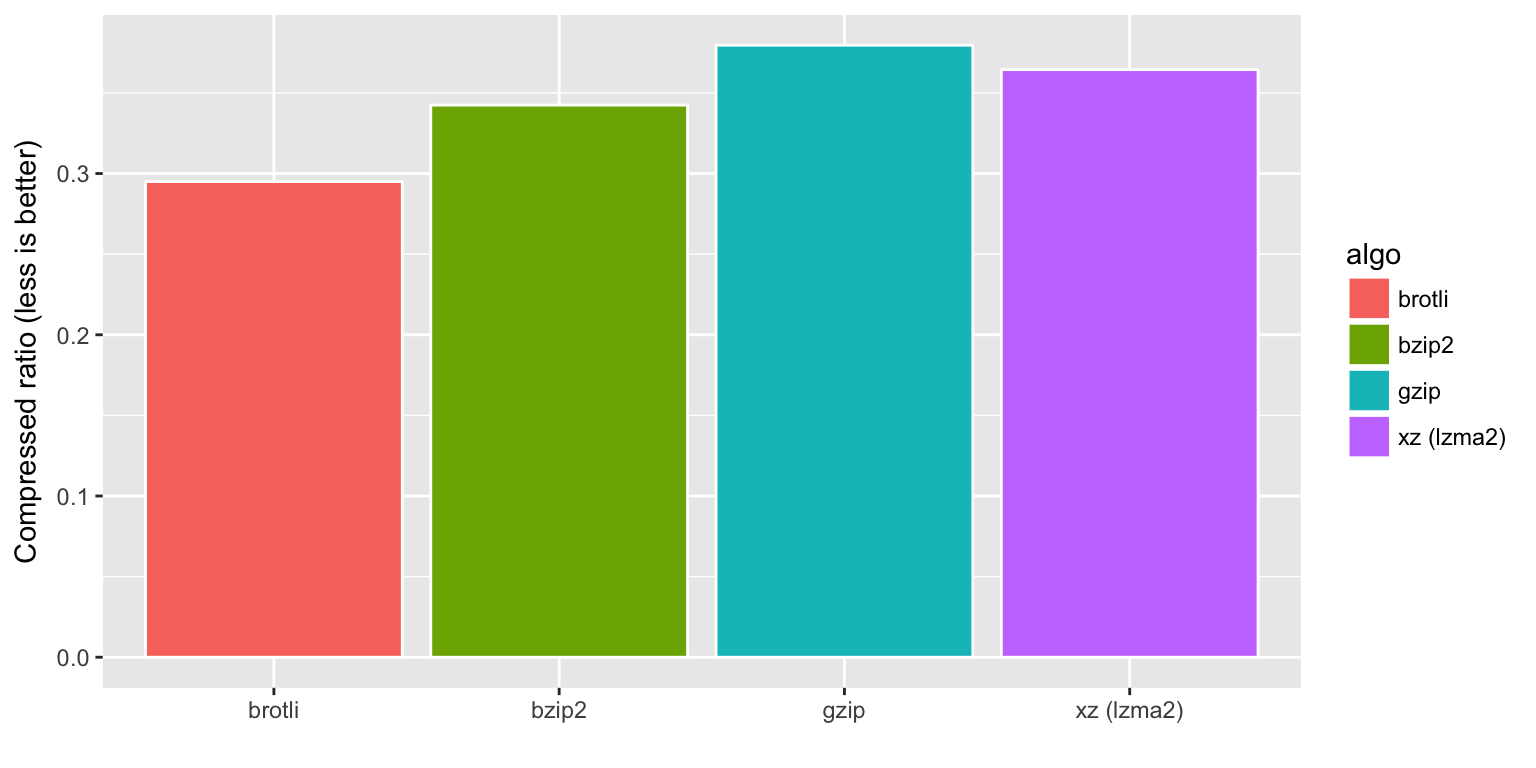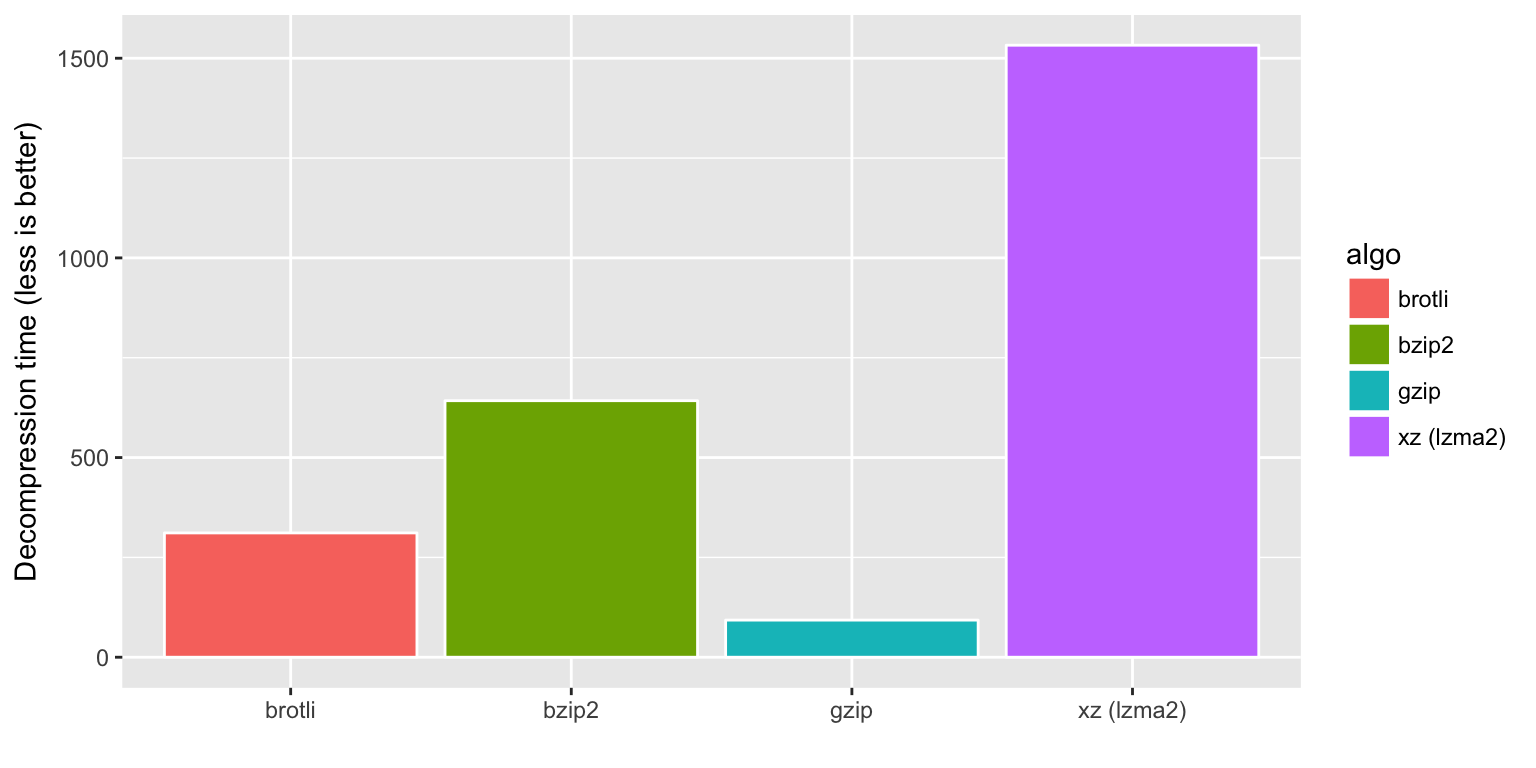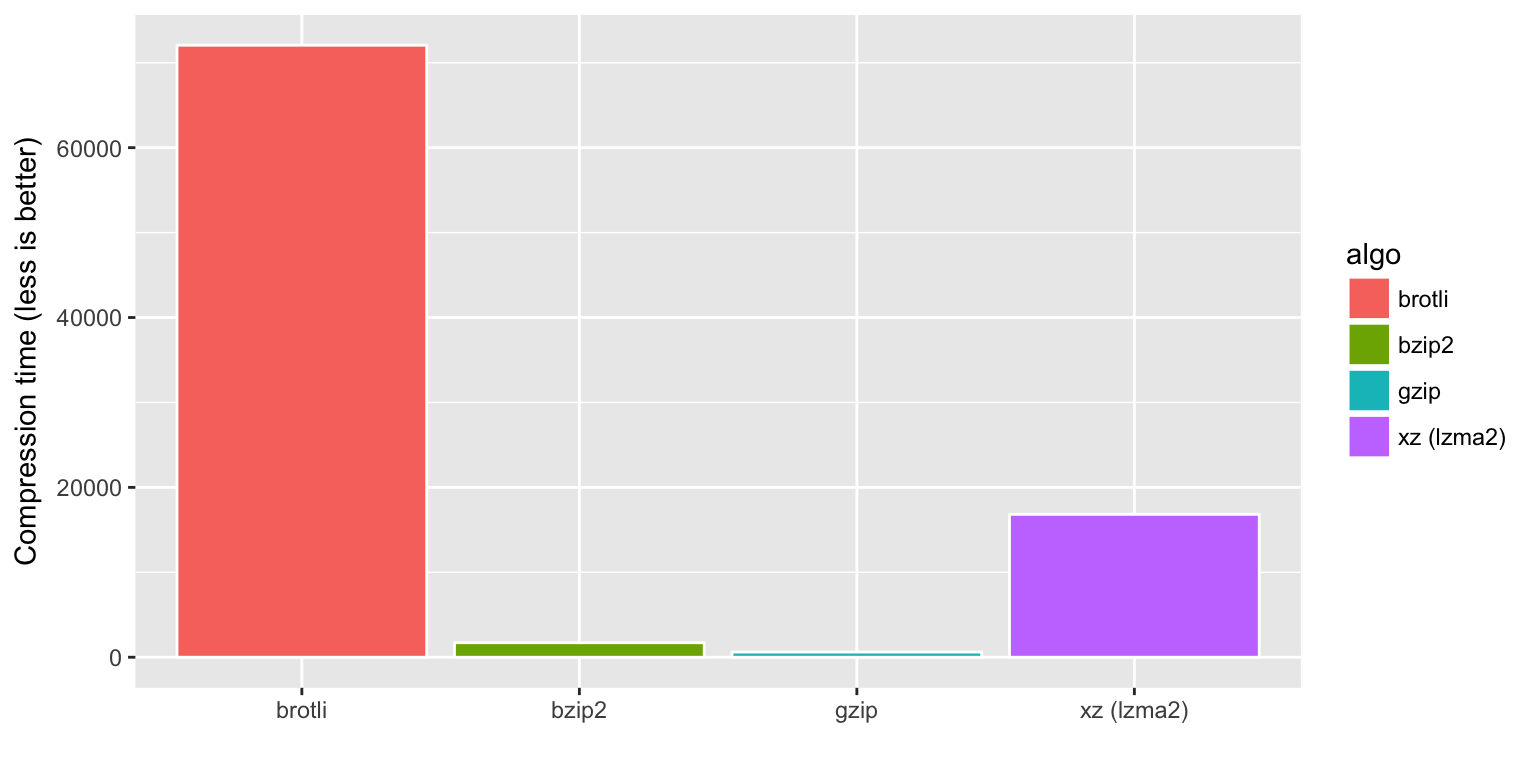Brotli is a new compression algorithm optimized for the web, in particular small text documents. Brotli decompression is at least as fast as for gzip while significantly improving the compression ratio. The price we pay is that compression is much slower than gzip. Brotli is therefore most effective for serving static content such as fonts and html pages.

Let’s benchmark some example text data from the COPYING file.

library(brotli)
library(ggplot2)

# Example data
myfile <- file.path(R.home(), "COPYING")
x <- readBin(myfile, raw(), file.info(myfile)$size) # The usual suspects y1 <- memCompress(x, "gzip") y2 <- memCompress(x, "bzip2") y3 <- memCompress(x, "xz") y4 <- brotli_compress(x) Confirm that all algorithms are indeed lossless: stopifnot(identical(x, memDecompress(y1, "gzip"))) stopifnot(identical(x, memDecompress(y2, "bzip2"))) stopifnot(identical(x, memDecompress(y3, "xz"))) stopifnot(identical(x, brotli_decompress(y4))) ## Compression ratio If we compare compression ratios, we can see Brotli significantly outperforms the competition for this example. # Combine data alldata <- data.frame ( algo = c("gzip", "bzip2", "xz (lzma2)", "brotli"), ratio = c(length(y1), length(y2), length(y3), length(y4)) / length(x) ) ggplot(alldata, aes(x = algo, fill = algo, y = ratio)) + geom_bar(color = "white", stat = "identity") + xlab("") + ylab("Compressed ratio (less is better)")## Decompression speed Perhaps the most important performance dimension for internet formats is decompression speed. Clients should be able to decompress quickly, even with limited resources such as on browsers and mobile devices. library(microbenchmark) bm <- microbenchmark( memDecompress(y1, "gzip"), memDecompress(y2, "bzip2"), memDecompress(y3, "xz"), brotli_decompress(y4), times = 1000 ) alldata$decompression <- summary(bm)$median ggplot(alldata, aes(x = algo, fill = algo, y = decompression)) + geom_bar(color = "white", stat = "identity") + xlab("") + ylab("Decompression time (less is better)")We see that brotli is very similar to gzip in decompression speed. We also see why bzip2 and xz have never replaced gzip as the standard compression method on the internet, even though they have better compression ratio: they are several times slower to decompress. ## Compression speed So far Brotli showed the best compression ratio, with decompression performance comparable to gzip. But there is no such thing as a free pastry in Switzerland. Here is the caveat: compressing data with brotli is complex and slow: library(microbenchmark) bm <- microbenchmark( memCompress(x, "gzip"), memCompress(x, "bzip2"), memCompress(x, "xz"), brotli_compress(x), times = 20 ) alldata$compression <- summary(bm)\$median
ggplot(alldata, aes(x = algo, fill = algo, y = compression)) +
geom_bar(color = "white", stat = "identity") +
xlab("") + ylab("Compression time (less is better)")Hence we can conclude that Brotli is mostly nice for clients, with decompression performance comparable to gzip while significantly improving the compression ratio. These are powerful properties for serving static content such as fonts and html pages.

However compression performance, at least for the current implementation, is considerably slower than gzip, which makes Brotli less suitable for on-the-fly compression in http servers or other data streams.